Next: Worked example 12.5: Mass Up: Orbital motion Previous: Worked example 12.3: Circular

## Worked example 12.4: Halley's comet

Question: The distance of closest approach of Halley's comet to the Sun is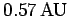. (1 AU is the mean Earth-Sun distance.) The greatest distance of the comet from the Sun is 35AU. The comet's speed at closest approach is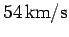. What is its speed when it is furthest from the Sun?

Answer: At perihelion and aphelion, the comet's velocity is perpendicular to its position vector from the Sun. Hence, at these two special points, the comet's angular momentum (around the Sun) takes the particularly simple form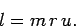Here,is the comet's mass,is its distance from the Sun, andis its speed. According to Kepler's second law, the comet orbits the Sun with constant angular momentum. Hence, we can write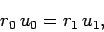where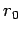and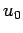are the perihelion distance and speed, respectively, and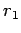and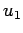are the corresponding quantities at aphelion. We are told that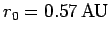,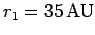, and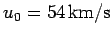. It follows that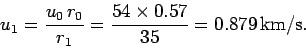Next: Worked example 12.5: Mass Up: Orbital motion Previous: Worked example 12.3: Circular
Richard Fitzpatrick 2006-02-02